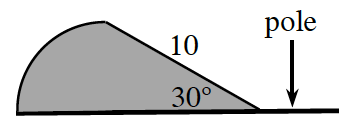### Home > APCALC > Chapter 1 > Lesson 1.4.2 > Problem1-152

1-152.

The shaded region at right represents a quarter circle combined with a right triangle “flag.”

1. Imagine rotating this flag about its “pole” and describe the resulting three-dimensional figure. Draw a picture of this figure on your paper.

2. Calculate the volume of the rotated flag.

Sum together the volumes of the cone and the half-sphere. Note that the radius is obtained by using the trigonometry rule for $30^\circ − 60^\circ − 90^\circ$ triangles.

$\text{The volume of a sphere is }\frac{4}{3}\pi r^{3},\text{ so the volume of half a}$

$\text{sphere would be half of that. The volume for a cone is }\frac{1}{3}\pi r^{2}h.$Use the eTool below to visualize the flag.
Click the link at right for the full version of the eTool: Calc 1-152 HW eTool